# How to Create Histograms in Excel 2016/2013/2010 for Mac and Windows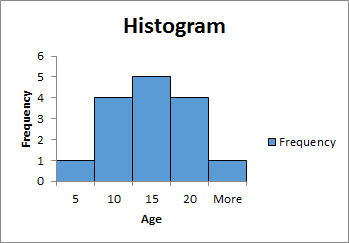A histogram is simply a bar graph that shows the occurrence of data intervals into a bin range. Or say it shows the frequency distributions in data.
A histogram may look like a column graph but it is not interpreted from the column's height. It is intrepreted from the area it covers.
A bin is defined for frequency distribution. It is a kind of grouping.
For example, if you want to know, in a school, how many students are of age 5 or below, how many are between 6-10, how many are between 11-15, how many are between 15-20 and how many are 20 or more. The histogram in excel is
the best way to analyze and visualize this data and get the answer.

Enough of the theory, let’s dig into a scenario.

## How to Make a Histogram on Excel 2016 Example:

Let's say that we have this data in excel.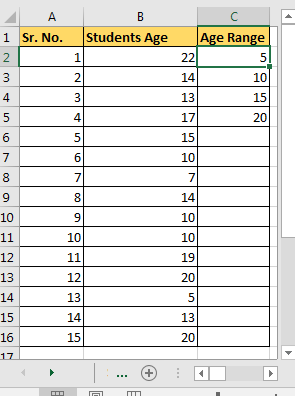• In Column A we have Sr. No.
• In Column B we have Age.
• In Column C we have the age range or say it's our excel histogram bin range. It shows that we want to know the number of students whose age is:

age<=5, 5<age<=10, 10<age<=15, 15<age<=20 and age>20. Simple, isn’t it? It is used to produce a frequency distribution.

Now, to plot a histogram chart in Excel 2016, we will use Data Analysis add-in. I assume you have read how to add data Analysis Add-In Excel for adding Data Analysis Toolpak. If you have already added it in, we can continue to our histogram tutorial.

## Make Histogram Using Data Analysis ToolPak

To create a histogram in Excel 2016/2013/2010 for Mac and Windows, follow these simple steps:

1. Go to the Data tab and click on Data Analysis.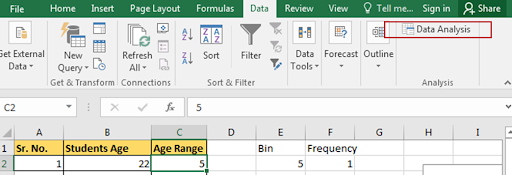1. Select Histogram in Data Analysis ToolPak Menu Dialog and hit the OK button.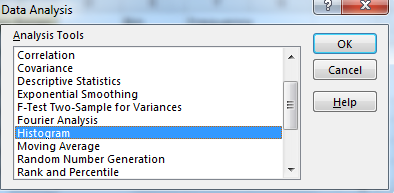1. In Input Range, select your data. In Bin Range select the interval range. Now if you have selected headers then check Labels, else leave it.

Select the output range. The place where you want to show your histogram in Excel worksheets. For this example, I have selected E13 on the same sheet.

Check Chart Output checkbox for Histogram chart.

Press that OK button on top. It will plot a histogram on the excel sheet.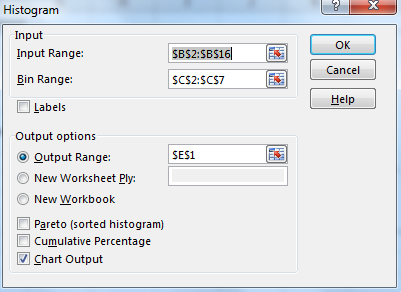1. Now we have created a histogram chart in Excel.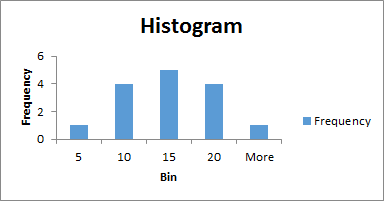We need to do a little bit of editing in this chart.

1. Select the bar and right-click. Select the format chart area. In Excel 2016 you will see this kind of menu. It is different in older versions.

Click on the little bars shown. Reduce the gap width to 0%.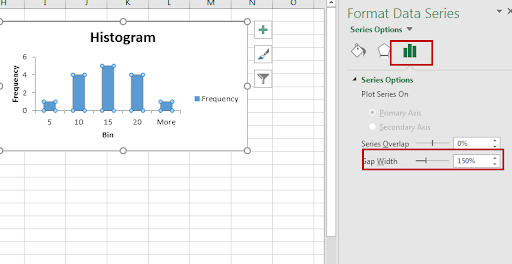1.  It's done. You can also add borders to the graph to look a little bit organized. You can learn how to format the chart beautifully in excel in 2016.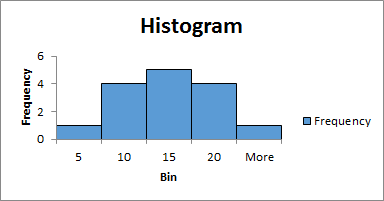So yeah guys. It is done. We can tell that most of the students are b/w age of 10-15 and 15-20 by just looking at this Excel Histogram chart.

## How to Create Histogram in Using Formula - Dynamic Histogram

Now the biggest problem with the above method of creating Histogram in Excel, that its static. It's good when you want to create a quick report but will be useless if your data changes from time to time. You can make this dynamic by using formula. Since it shows frequency distribution, we can use the FREQUENCY function of excel, for making excel histogram charts. Let's see how…

So again we have that same data of students and same bin range. Now follow these steps to create a dynamic Histogram in excel 2010, 2013, and 2016 and above.

1. Write heading as Frequency in the adjacent column of the bin range and select all cell adjacent to bin range. Select one extra cell to then bin range as shown in the image below. This very important.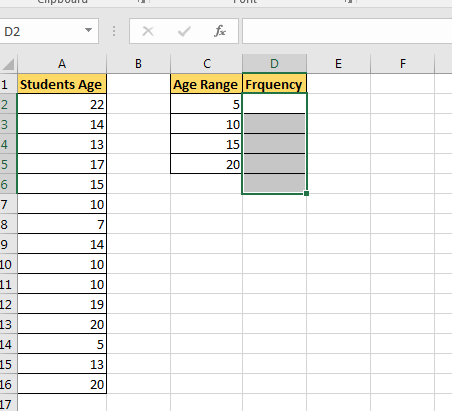1. Now click on the formula bar and write this Frequency formula. As data array, select A2:A16 and as bin range, select C2:C5. Press Control+Shift+Enter. Yup, we need an array formula here. Make sure that you have selected a cell extra then bin range. This is for values found in more than the largest bin value. You can name it More or >20.
 {=FREQUENCY(A2:A16,C2:C5)}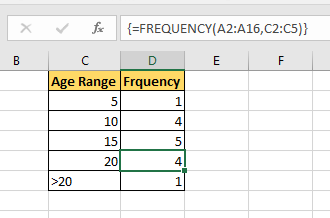1. Now select this bin range and frequency and goto insert tab.
2. Goto chart section and select column chart. You can use a bar chart or line chart, but that's not the traditional histogram.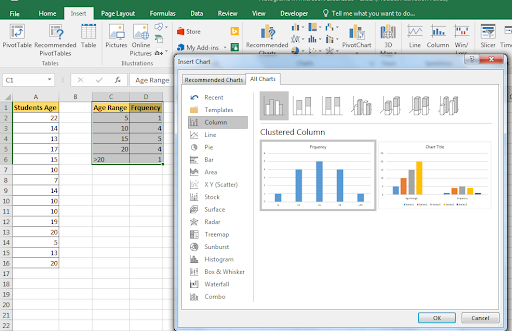1. You have your dynamic histogram chart created in excel. Now, whenever you’ll change the data in excel it will change accordingly. It's best to use named ranges for the dynamic histogram in excel.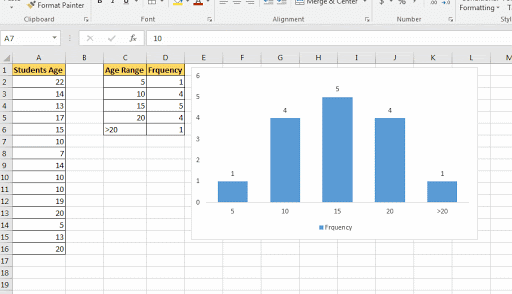This chart is version independent. You can create this Histogram chart in Excel 2010/2013/2016/2019/365 and any upcoming since it is based on formula instead of any version-specific feature.

## How to Create Histogram Directly From Charts In Excel 2016

In Excel 2013 and 2016, we can directly create a histogram chart by using predefined histogram template in excel. It is useful when you don’t have defined bins for a histogram.

Steps to create histogram directly from the charts.

1. Select data.
2. Go to Insert Tab. Locate the chart section.
3. Click on Recommended Charts.
4. Click on All Charts.
5. On the left Histogram. 4th from Bottom. There are two options. Histogram and Pareto. Select Histogram and click ok.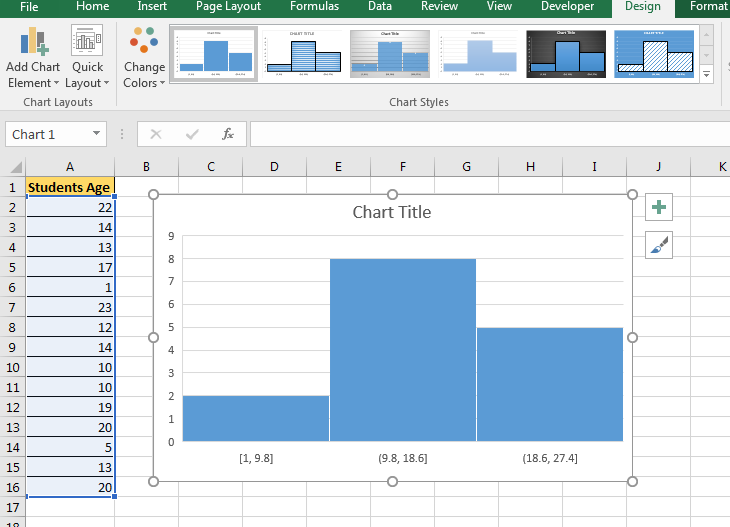Now you have your, Histogram chart. It has predefined bins. You can customise to some extent. You can see it created 3 bins [1-9.8], (9.8-18.6], and (18.6, 27.4]. If you don want this then you can edit it.

To edit this histogram

• Right-click on bins and click Format Axis.
• You can now define your bin numbers or bin width
• There are several other options available to customize the Histogram in  Excel.

I personally don’t prefer this chart. But its useful when i don’t have a bin range.

So we learned how to plot histogram in excel 2016. The histogram in Excel 2013 has the same procedure. Same case for older versions of Excel. Only the formatting options have changed.
Related Articles:

How to use the Waterfall Chart in Excel

4 Creative Target Vs Achievement Charts in Excel

How to use the Pareto Chart and Analysis In Microsoft Excel

How to do Regressions in Excel

Popular Articles:

50 Excel Shortcuts to Increase Your Productivity

How to use the VLOOKUP Function in Excel

How to use the COUNTIF function in Excel

How to use the SUMIF Function in Excel

Terms and Conditions of use

The applications/code on this site are distributed as is and without warranties or liability. In no event shall the owner of the copyrights, or the authors of the applications/code be liable for any loss of profit, any problems or any damage resulting from the use or evaluation of the applications/code.